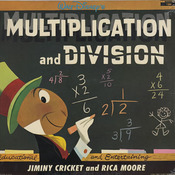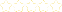+# Multiplication and Division

Owner: Rachel Casto
##### Description:
Operations and Algebraic Thinking ⎯ 4.OA  A. Use the four operations with whole numbers to solve problems. 3. Solve multistep word problems posed with whole numbers and having whole-number answers using the four operations, including problems in which remainders must be interpreted. Represent these problems using equations with a letter standing for the unknown quantity. Assess the reasonableness of answers using mental computation and estimation strategies including rounding. Number and Operations in Base Ten 2 ⎯ 4.NBT  B. Use place value understanding and properties of operations to perform multi-digit arithmetic. 5. Multiply a whole number of up to four digits by a one-digit whole number, and multiply two two-digit numbers, using strategies based on place value and the properties of operations. Illustrate and explain the calculation by using equations, rectangular arrays, and/or area models. 6. Find whole-number quotients and remainders with up to four-digit dividends and one-digit divisors, using strategies based on place value, the properties of operations, and/or the relationship between multiplication and division. Illustrate and explain the calculation by using equations, rectangular arrays, and/or area models.   NOTE: 2 Grade 4 expectations in this domain are limited to whole numbers less than or equal to 1,000,000. Measurement and Data ⎯ 4.MD  A. Solve problems involving measurement and conversion of measurements from a larger unit to a smaller unit.  3. Apply the area and perimeter formulas for rectangles in real world and mathematical problems. For example, find the width of a rectangular room given the area of the flooring and the length, by viewing the area formula as a multiplication equation with an unknown factor.
4 Tutorials in this Playlist

#### Multiplication

Author:
Rating:(0)

#### Division

Author:
Rating:(1)

#### Perimeter-AROUND

Author:
Rating:(0)

#### Area-INSIDE

Author:
Rating:(0)